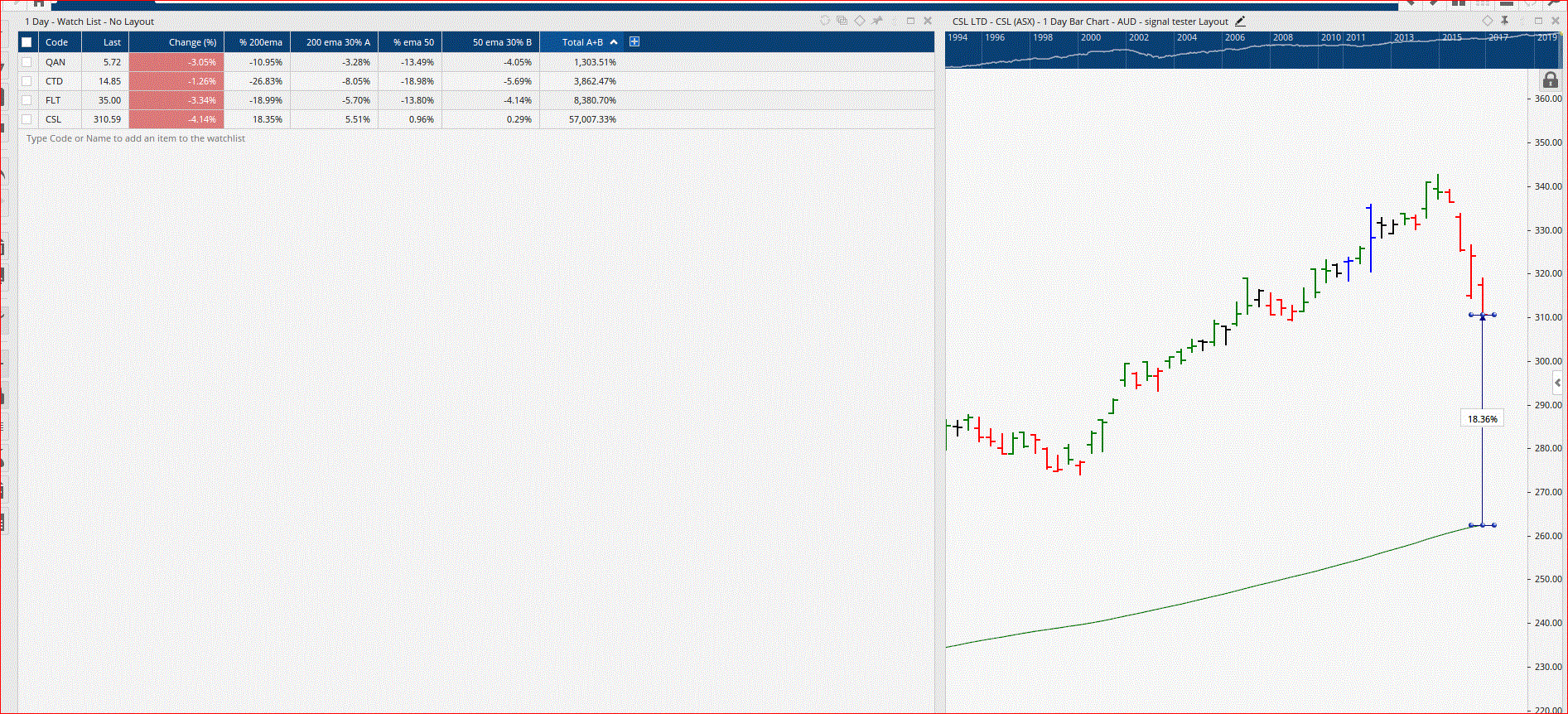Select Page

# Sum the results of variables

Optuma Forums Optuma Scripting Sum the results of variables

Viewing 5 posts - 1 through 5 (of 5 total)
• Author
Posts
• #57083

I’m trying to sum the results of 2 variables to use in a ranking system. The script of each variable is valid when run individually but when the ‘v’ for variable is added the script is no longer valid. What am I missing?

V1=HI200=MA(BARS=200, STYLE=Exponential, CALC=Close); (CLOSE() – HI200)/HI200*30;
V2=ROC(BARS=120)*30;
// sum the results of v1 + v2
sum V1+V2

I then want want to sum the results of v1 +v2, not sure if this is possible.

Thanks Peter

#57085

Hi Peter,

You have already called the first variable HL200. In fact there is also 2 semi-colons in that first line too – something looks like you have cut and pasted into the wrong location. Once you have sorted that out, the sum will just be V1+v2

Hope that helps

Mathew

#57155

Still not working. The problem is with the moving average script

HI50=MA(BARS=50, STYLE=Exponential, CALC=Close);
(CLOSE() – HI50)/HI50*30/100

This script is valid in its own right, but when I add the next criteria the script becomes invalid. Once again on its own the script is valid

HI200=MA(BARS=200, STYLE=Exponential, CALC=Close);
(CLOSE() – HI200)/HI200*30/100

So the final script is

HI50=MA(BARS=50, STYLE=Exponential, CALC=Close);
(CLOSE() – HI50)/HI50*30/100;
HI200=MA(BARS=200, STYLE=Exponential, CALC=Close);
(CLOSE()-HI200)/HI200*30/100;
HI50+HI200
Summing multiply ROC values is not causing any problems. My aim is to sum the values of the moving averages by weighting in % terms.

Once again each individual script is giving the correct outcome when placed in a watch list.

Where am I going wrong?

Thanks Peter

#57157

Hi Peter,

You haven’t assigned a variable name to the 2nd or 4th lines of your completed script.

Your final line is summing lines 1 and 3, so the other lines aren’t required.  If you do plan on using them in a more expanded script you need to give them a variable assignment…

Once you do the script becomes valid.

#57183

Ok the script is now working but still not giving the result I’m looking for. I’m wanting to sum the values of the two moving averages. Using CSL as the test,

the values are A 30% of 200 ema = 5.51 and B 30% of 50 ema = 0.29% total =5.8.  The script is giving this value as 57007.33%. I’m lost how to make the script give the correct answer. I have a script totalling numerous ROC values correctly. Where to next?Viewing 5 posts - 1 through 5 (of 5 total)
• You must be logged in to reply to this topic.